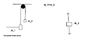# Atwood machine at rest and released

• Poetria

## Homework Statement

In the image shown, two blocks hang on a pulley. Assume that m1 is the more massive block, and that the pulley is massless and frictionless.

Take the initial case to be the instant just after m1 is released from rest, and the final case to be the instant just before m2 hits the pulley. Compare the magnitudes of the quantities below at those times.

The initial tension on m1 versus the final tension on m1.
The initial tension on m1 versus the force of gravity on m2.
The initial tension on m2 versus the final tension on m1.
The initial tension on m2 versus the force of gravity on m2

T-m_2 = m_2*a

m_1-T=m_1*a

## The Attempt at a Solution

_
I tried to figure out the initial tension on m_1. I thought it is m_2*g but this is wrong. I guess m_2*g is the tension of the whole string. Am I right?
This initial mistake caused the other.

#### Attachments

•Atwood machine.png
2.2 KB · Views: 488
I tried to figure out the initial tension on m_1. I thought it is m_2*g but this is wrong. I guess m_2*g is the tension of the whole string. Am I right?
Nope. The instant that m1 is released both masses begin to move according to the forces acting. You need to draw free-body diagrams for both masses and write expressions for the acceleration of each that include the force due to the tension in the string. Solve for the tension.

What can you say about the tension in the string on either side of the pulley? (Remember: thee pulley is massless and frictionless). How about the accelerations of the blocks, how are they related?

•Poetria
If the tension is the same on either side
The initial tension on m1 versus the force of gravity on m2.
and for
The initial tension on m2 versus the force of gravity on m2

would be the same, i.e. 'greater' because:
T=m_2*a+m_2*g

The acclerations of the two masses have the same magnitude but the opposite directions - down for the mass 1 and up for the mass 2.
Hm, is the tension the same on either side?

I have prepared FBDs.
Your FBD's look fine. And yes, the tensions are the same since the pulley can have no effect (massless, frictionless) other than change the direction of the string.

Can you solve for an expression for the tension? It will help to answer the questions.

•Poetria
If the tension is the same on either side
The initial tension on m1 versus the force of gravity on m2.
and for
The initial tension on m2 versus the force of gravity on m2

would be the same, i.e. 'greater' because:
T=m_2*a+m_2*g
Sure. I don't know if this is a "one word answer" problem or if you need to develop your argument a bit more, but you have reached the right conclusion.

•Poetria
Yes, this is one-word-answer problem but I would prefer to understand it more deeply. I am really grateful for your help. :)

So I combined the equations from the previous posts

m_1*g-m_1*a=m_2*g+m_2*a

m_1*g-m_2*g=m_2*a+m_1*a

a=(m_1*g-m_2*g)/(m_2+m_1)

And by substitution m_1*a=m_1*g-T:

m_1*((m_1*g-m_2*g)/(m_2+m_1))=m_1*g-T

T=(2*g*m_1*m_2)/(m_1+m_2)

Is this the equation you have asked for?

If so in the two cases:
The initial tension on m1 versus the final tension on m1.
The initial tension on m2 versus the final tension on m1.

the answer would be 'equal' because g doesn't change of course and the two masses stay the same.

Yes, this is one-word-answer problem but I would prefer to understand it more deeply. I am really grateful for your help. :)

So I combined the equations from the previous posts

m_1*g-m_1*a=m_2*g+m_2*a

m_1*g-m_2*g=m_2*a+m_1*a

a=(m_1*g-m_2*g)/(m_2+m_1)

And by substitution m_1*a=m_1*g-T:

m_1*((m_1*g-m_2*g)/(m_2+m_1))=m_1*g-T

T=(2*g*m_1*m_2)/(m_1+m_2)

Is this the equation you have asked for?
Yes. Nicely done.
If so in the two cases:
The initial tension on m1 versus the final tension on m1.
The initial tension on m2 versus the final tension on m1.

the answer would be 'equal' because g doesn't change of course and the two masses stay the same.
Yes, and a good argument too.

•Poetria
Many thanks :) :)